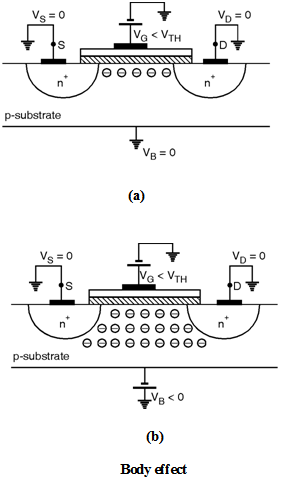Home > Digital CMOS Design > Non Ideal Effects > Body Effect

Body-Effect | Digital-CMOS-Design

Body Effect :

In I-V analysis we assumed that the bulk and source of transistor were tied to ground, what happens if the bulk voltage of NMOS is drops below the source voltage ?

To understand this effect suppose VS = 0 and VD = 0 and VG is somewhat less than VTH so that depletion region is formed under the gate but inversion channel does not exist as shown in Figure.

As VB becomes more negative (i.e. VB < VS where VS = 0) more holes are attracted to the substrate connection leaving a larger negatively charged ions behind i.e. the depletion region becomes wider as shown in Figure.

As we know that the threshold voltage is a function of the total charge in the depletion region (i.e. Qdep). Thus as the body voltage VB drops then depletion charge (Qdep) increases which increases the threshold voltage (VTH). This effect is called as the body effect or back gate effect.When the body bias voltage VSB is applied between source and body the surface potential required for strong inversion is increased and becomes |2 F + VSB|. The charge stored in the depletion region can now be expressed as :

Qdep = 2q NA Si | 2 f + VSB |

From the preceeding discussions it is clear that VSB has an impact on the threshold voltage.

Thus the threshold voltage with body bias can be expressed as :

VTH = VTHO +  (| 2 F + VSB |  | 2 F |

Where VTHO is given by,

 = 2q Si NsubCox is the body effect coefficient.